alonsoformula In Spanish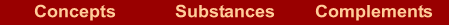In the formulae

The element that is written on the left is the most electropositive (the one that has positive oxidation number), and on the right you write the most electronegative (the one that has negative oxidation number). Normally, these positions coincide with the place where the elements appear in the periodic table, the electropositive elements on the left and the electronegative ones on the right.

How many atoms of every element are there in a formula?

In every neutral chemical compound, the sum of the results when we multiply the oxidation number by the number of atoms of every element must be zero. We can calculate how many atoms of every element must be so that the compound be electrically neutral.

What compounds will give the atoms A and B with different oxidation numbers?

 Atom A Atom B Atoms of each so that the compound be neutral Formula Example A+I B-I (+1)+(-1)=0 AB Na+      Cl- NaCl A+II B-I (+2)+2(-1)=0 AB2 Ca+2      Br- CaBr2 A+II B-III 3(+2)+2(-3)=0 A3B2 Mg+2      N-3 Mg3N2 A+IV B-II (+4)+2(-2)=0 AB2 Pb+4      O-2 PbO2

In the names

In first place we mention the element that is on the left in the formula and next the element that is on the right.

Binary ionic compounds: the name is formed by two words.

- First word: Name of the element on the left , or less electronegative, with its oxidation number if it is not invariable.
- Second word: Stem of the element on the right, or more electronegative, plus the suffix -ide.

 KBr Potassium bromide CaO Calcium oxide CuCl2 Copper(II) chloride

Binary covalent compounds : the name is formed by two words.

- First word: Name of the element on the left , or less electronegative, with a Greek prefix that indicates the number of atoms.
- Second word: Stem of the element on the right, or the most electronegative, plus the suffix -ide, and with a Greek prefix that indicates the number of atoms.

 CO Carbon monoxide N2O5 Dinitrogen pentaoxide PCl3 Phosphorous trichloride

Home | Introduction | Oxidation number | Mechanics | Kind of substances | Elementary substances | Metal oxides | Nonmetal oxides | Metal-nonmetal compounds | Nonmetal-nonmetal compounds | Metal hydrides | Hydracids | H with nonmetal | Hydroxides | Oxyacids | Oxysalts | Acid salts | Exercises | Periodic Table | Links
Author: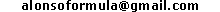WWW.ALONSOFORMULA.COM Formulación InorgánicaFormulación OrgánicaFormulación Inorgánica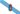Formulación OrgánicaFormulació Inorgánica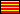Formulació OrgánicaEzorganikoaren Formulazioa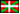Nomenclature of Inorganic Q.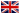Física y Química de ESOFísica e Química de ESOFQ de 1º de BachilleratoFQ de 1º de BacharelatoQuímica de 2º de BachilleratoPrácticas de Química